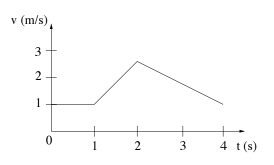# Problem: In the graph shown below, velocity is plotted as a function of time for an object traveling in a straight line. A) The object moves backward from 2 to 4 seconds. B) The object is at rest from 0 to 1 seconds. C) From 1 to 2 seconds the acceleration is largest. D) The object returns to where it started after 4 seconds.

###### FREE Expert Solution
94% (326 ratings)
###### Problem Details

In the graph shown below, velocity is plotted as a function of time for an object traveling in a straight line.

A) The object moves backward from 2 to 4 seconds.

B) The object is at rest from 0 to 1 seconds.

C) From 1 to 2 seconds the acceleration is largest.

D) The object returns to where it started after 4 seconds.Frequently Asked Questions

What scientific concept do you need to know in order to solve this problem?

Our tutors have indicated that to solve this problem you will need to apply the Velocity-Time Graphs & Acceleration concept. You can view video lessons to learn Velocity-Time Graphs & Acceleration. Or if you need more Velocity-Time Graphs & Acceleration practice, you can also practice Velocity-Time Graphs & Acceleration practice problems.

How long does this problem take to solve?

Our expert Physics tutor, Jeffery took 2 minutes and 19 seconds to solve this problem. You can follow their steps in the video explanation above.

What professor is this problem relevant for?

Based on our data, we think this problem is relevant for Professor Kezerashvili's class at BMCC CUNY.